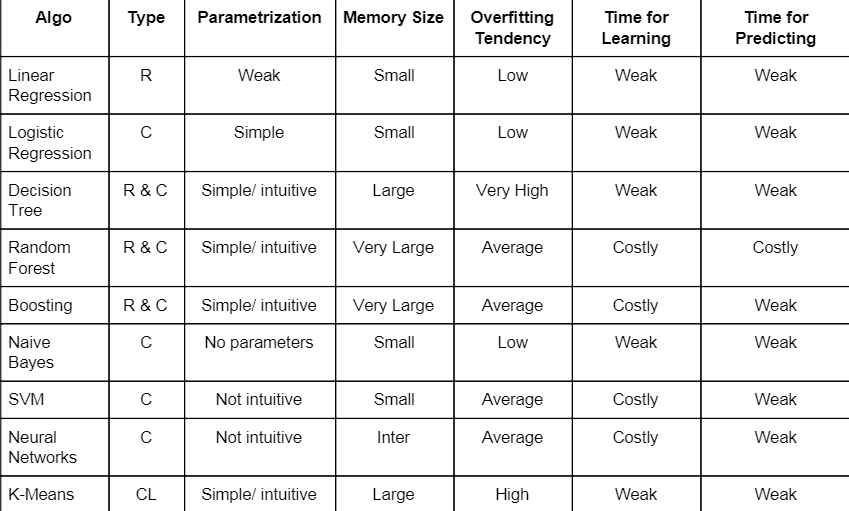# Machine Learning Algorithms

Original article was published on Artificial Intelligence on Medium# Machine Learning Algorithms

Machine learning is a branch of artificial intelligence that allows computer systems to learn directly from examples, data, and experience.It has many algorithms and unfortunately we are unable to select the right algorithm for the right problem.Following are the factors which help us to choose the correct algorithm

## Factors help to choose algorithm

1-Type of algorithm

2-Parametrization

3-Memory size

4-Overfitting tendency

5-Time of learning

6-Time of predicting

## Type of algorithm

1.Regression

It is a technique used to predict dependent variable in set of independent variable.The algorithms which come under regression are

1-Linear Regression

2-Decision Tree

3-Random Forest

4-Boosting

2 . Classification

It is a technique used for approximating a mapping function (f) from input variables (X) to discrete output variables (y).The algorithms which come under classification are

1-Logistic Regression

2-Naive Bayes

3-SVM

4-Neural Networks

5-Decision Tree

6-Random Forest

6-Boosting

3. Clustering

It is a technique for dividing the population or data points into a number of groups such that data points in the same groups are more similar to other data points in the same group and dissimilar to the data points in other groups. K-means is important algorithm used for clustering

Note Decision tree, Random forest and Boosting are the algorithm which can be used for both classification and regression

## Parametrization

Parameters are key to machine learning algorithms. They are the part of the model that is learned from historical training data.Parameters are key to machine learning algorithms. They are the part of the model that is learned from historical training data.We are classifying our parameters as

1-No parameters

2-Weak

3-Simple/Intuitive

4-Not Intuitive

## Memory Size

It is the space we need to store our data and variables.Researchers are struggling with the limited memory bandwidth of the DRAM devices that have to be used by today’s systems to store the huge amounts of weights and activations in DNNs.GPUs and other machines designed for matrix algebra also suffer another memory multiplier on either the weights and activations of a neural network. We are classifying our memory size required as

1-Small

2-Large

3-Very Large

## Overfitting Tendency

When a model tries to predict a trend in data that is too noisy. Overfitting is the result of an overly complex model with too many parameters. A model that is overfitted is inaccurate because the trend does not reflect the reality of the data.There are many techniques that can be use to mitigate overfitting, including cross-validation, regularization, early stopping, pruning, Bayesian priors, dropout and model comparison. We are classifying Overfitting tendency as

1-Low

2-Average

3-High

4-Very high

## Time for Learning

Time for learning is time associate with training of dataset.It varies with size of data and algorithm we are using in that. We are classifying time for learning as

1-Weak

2-Costly

3-Very Costly

## Time for Predicting

Time of predicting is time associate with testing of dataset.It varies with size of data and algorithm we are using in that.We are classifying time for learning as

1-Weak

2-Costly